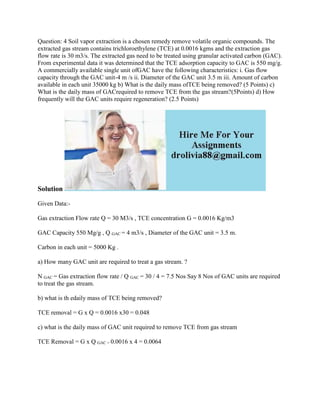Se está descargando tu SlideShare. ×

# Question- 4 Soil vapor extraction is a chosen remedy remove volatile o (1).docx

Anuncio
Anuncio
Anuncio
Anuncio
Anuncio
Anuncio
Anuncio
Anuncio
Anuncio
Anuncio
AnuncioCargando en…3
×

1 de 2 Anuncio

# Question- 4 Soil vapor extraction is a chosen remedy remove volatile o (1).docx

Question: 4 Soil vapor extraction is a chosen remedy remove volatile organic compounds. The extracted gas stream contains trichloroethylene (TCE) at 0.0016 kgms and the extraction gas flow rate is 30 m3/s. The extracted gas need to be treated using granular activated carbon (GAC). From experimental data it was determined that the TCE adsorption capacity to GAC is 550 mg/g. A commercially available single unit ofGAC have the following characteristics: i. Gas flow capacity through the GAC unit-4 m /s ii. Diameter of the GAC unit 3.5 m iii. Amount of carbon available in each unit 35000 kg b) What is the daily mass ofTCE being removed? (5 Points) c) What is the daily mass of GACrequired to remove TCE from the gas stream?(5Points) d) How frequently will the GAC units require regeneration? (2.5 Points)
Solution
Given Data:-
Gas extraction Flow rate Q = 30 M3/s , TCE concentration G = 0.0016 Kg/m3
GAC Capacity 550 Mg/g , Q GAC = 4 m3/s , Diameter of the GAC unit = 3.5 m.
Carbon in each unit = 5000 Kg .
a) How many GAC unit are required to treat a gas stream. ?
N GAC = Gas extraction flow rate / Q GAC = 30 / 4 = 7.5 Nos Say 8 Nos of GAC units are required to treat the gas stream.
b) what is th edaily mass of TCE being removed?
TCE removal = G x Q = 0.0016 x30 = 0.048
c) what is the daily mass of GAC unit required to remove TCE from gas stream
TCE Removal = G x Q GAC = 0.0016 x 4 = 0.0064
.

Question: 4 Soil vapor extraction is a chosen remedy remove volatile organic compounds. The extracted gas stream contains trichloroethylene (TCE) at 0.0016 kgms and the extraction gas flow rate is 30 m3/s. The extracted gas need to be treated using granular activated carbon (GAC). From experimental data it was determined that the TCE adsorption capacity to GAC is 550 mg/g. A commercially available single unit ofGAC have the following characteristics: i. Gas flow capacity through the GAC unit-4 m /s ii. Diameter of the GAC unit 3.5 m iii. Amount of carbon available in each unit 35000 kg b) What is the daily mass ofTCE being removed? (5 Points) c) What is the daily mass of GACrequired to remove TCE from the gas stream?(5Points) d) How frequently will the GAC units require regeneration? (2.5 Points)
Solution
Given Data:-
Gas extraction Flow rate Q = 30 M3/s , TCE concentration G = 0.0016 Kg/m3
GAC Capacity 550 Mg/g , Q GAC = 4 m3/s , Diameter of the GAC unit = 3.5 m.
Carbon in each unit = 5000 Kg .
a) How many GAC unit are required to treat a gas stream. ?
N GAC = Gas extraction flow rate / Q GAC = 30 / 4 = 7.5 Nos Say 8 Nos of GAC units are required to treat the gas stream.
b) what is th edaily mass of TCE being removed?
TCE removal = G x Q = 0.0016 x30 = 0.048
c) what is the daily mass of GAC unit required to remove TCE from gas stream
TCE Removal = G x Q GAC = 0.0016 x 4 = 0.0064
.

Anuncio
Anuncio

### Question- 4 Soil vapor extraction is a chosen remedy remove volatile o (1).docx

1. 1. Question: 4 Soil vapor extraction is a chosen remedy remove volatile organic compounds. The extracted gas stream contains trichloroethylene (TCE) at 0.0016 kgms and the extraction gas flow rate is 30 m3/s. The extracted gas need to be treated using granular activated carbon (GAC). From experimental data it was determined that the TCE adsorption capacity to GAC is 550 mg/g. A commercially available single unit ofGAC have the following characteristics: i. Gas flow capacity through the GAC unit-4 m /s ii. Diameter of the GAC unit 3.5 m iii. Amount of carbon available in each unit 35000 kg b) What is the daily mass ofTCE being removed? (5 Points) c) What is the daily mass of GACrequired to remove TCE from the gas stream?(5Points) d) How frequently will the GAC units require regeneration? (2.5 Points) Solution Given Data:- Gas extraction Flow rate Q = 30 M3/s , TCE concentration G = 0.0016 Kg/m3 GAC Capacity 550 Mg/g , Q GAC = 4 m3/s , Diameter of the GAC unit = 3.5 m. Carbon in each unit = 5000 Kg . a) How many GAC unit are required to treat a gas stream. ? N GAC = Gas extraction flow rate / Q GAC = 30 / 4 = 7.5 Nos Say 8 Nos of GAC units are required to treat the gas stream. b) what is th edaily mass of TCE being removed? TCE removal = G x Q = 0.0016 x30 = 0.048 c) what is the daily mass of GAC unit required to remove TCE from gas stream TCE Removal = G x Q GAC = 0.0016 x 4 = 0.0064